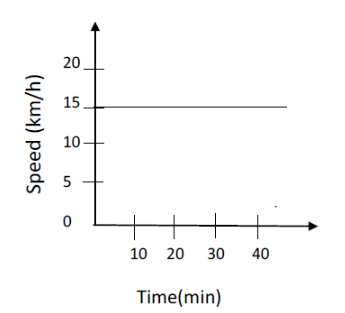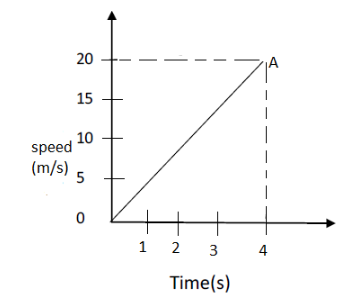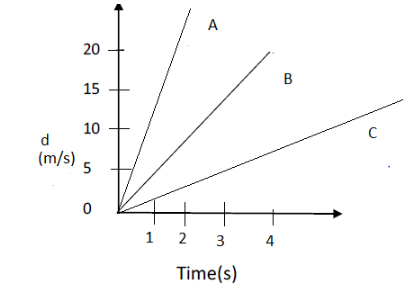# Question Bank on Motion for Class 9 physics

In this page we have Question Bank on Motion for Class 9 physics . (a) True and false (b) Short questions
(c) Graphical questions
Hope you like them and do not forget to like , social share and comment at the end of the page.

## True and False

Question 1
True and false questions
a. Displacement cannot be zero
b. Average speed= Total distance/time
c. Average velocity = Total displacement /time
d. slope of distance-time graph indicates the speed
e. It is possible to have Object moving with uniform speed but variable acceleration.
f. It is possible to have Object moving with uniform velocity but non-uniform acceleration.

Question 2
A person walks along the sides of a square field. Each side is 10m long. What is the maximum magnitude of displacement of the person in any time interval?

Question 3
A bullet of mass 10g travelling horizontally with a velocity of 150m/s strikes a stationary wooden block  and comes to the rest in 0.03 s. calculate the distance of penetration of the bullet into the block. Also calculate the magnitude of the force exerted by the wooden block on the bullet.

Question 4.
(a) A body starts from rest and gains a velocity of 6 m/s in 2s of time. Find the acceleration of the body and its average velocity for the given time:
(b) A force of 24 N produces an acceleration of 3m/s2 on a body of mass m1 and an acceleration of 2m/s2 on a body of mass m2. What acceleration does it produce when applied on a combination of the two bodies?

Question 5.
A car is accelerated uniformly from 36 km per hour to 90 km per hour in 3 seconds. Calculate: (a) The acceleration of car   (b)The distance covered by the car in that time.

Question 6.
Abdul while driving to school, computes the average speed for his trip to be 20 km/h. on his return trio along th same route, there is less traffic and the average speed is 30km/h. what s the average speed for abdul’s trip?

Question 7.
A scooter is moving with an initial velocity of 90 km/hr and it takes 10s to stop it, after the brakes are applied. Calculate the force exerted by the brakes on the scooter if its mass along with the rider is 200 kg.What would be the force if the mass is halved?

Question 8
A swimmer swims 90m long pool. He covers the distance of 180m by swimming from one end to other end back along the same path. If he covers the first 90m at speed of 2m/s, then how fast he swim so that his average speed is 3m/s?

Question 9
a. Write the difference between Distance and displacement
b. Write the difference between Uniform and Non uniform Motion

Question 10
Lori moves 4m due east and then 3m due west.
1. What is the distance covered by the Lori?
2. What is its displacement

Question 11
A car moves at a speed of 40km/h, It is stopped by applying brakes which produces a uniform acceleration of -0.6m/s2. How much distance will the vehicle move before coming to stop?

## Graphical Questions

Question 12.
A motorcyclist riding motorcycle A who is travelling at 36 km/h applies the brakes and stops the motorcycle in 10 s. Another motorcyclist of motorcycle B who is travelling at 18 km/h applies the breaks and stops the motorcycle in 20s. Plot speed-time graph for the two motorcycles. Which of the two motorcycles traveled farther before it comes to a stop?

Question 13
Below Figure represents the speed time graph for a particle. Find the distance covered by the particle
a. between t= 30min. and t= 40min.
b. between t=10 min and t=40 min
c. what is the speed of the particleVery Important question

Question 14
A particle starts from rest and moves with a uniform acceleration of 5m/s2 for 10s and then it moves with a constant velocity for 4s. Later it slows down and comes to rest in 5s. Draw the velocity graph for the motion of the body and answer the following questions:
a. What is the maximum velocity attended by the body?
b. What is the distance travelled during this period of acceleration?
c. What is distance travelled when the body was moving with constant velocity?
d. What is the retardation of the body while slowing down?
e. What is the distance travelled by retarding?
f. What is the total distance travelled?

Question 15
Two object are thrown vertically upwards simultaneously with their velocities x and y respectively. Prove that
a. The heights reached by them would be in the ratio of x2: y2
b. The time taken to reach the maximum height would be in the ratio of x:y
(Assume upward acceleration is –g and downward acceleration to be +g).

Question 16
Find the distance covered by a particle during the time interval t=0 and t=4s for which the speed time graph is shown in figureQuestion 17
Figure shows distance time graph of three objects A ,B and C
a. which object is moving with a greater speed
b. which object is moving with slowest speedQuestion 18
Marc runs from one end to the other end of a semicircular track whose radius is 140m. What is the distance covered by the marc and what is his displacement?

Question 19
A jogger moves 500m in 2 minutes and next 1000m in 30s on the same straight path. What is his average speed and average velocity?

Question 20

A big truck moving along a straight line at a speed of 54km/hr stop in 5s after the breaks are applied.
1. Find the acceleration, assuming it to constant.
2. Plot the graph of speed versus time.
3. Using the graph. Find the distance covered by the car after the brakes are applied?

## Summary

This Question Bank on Motion for Class 9 physics with answers is prepared keeping in mind the latest syllabus of CBSE . This has been designed in a way to improve the academic performance of the students. If you find mistakes , please do provide the feedback on the mail.